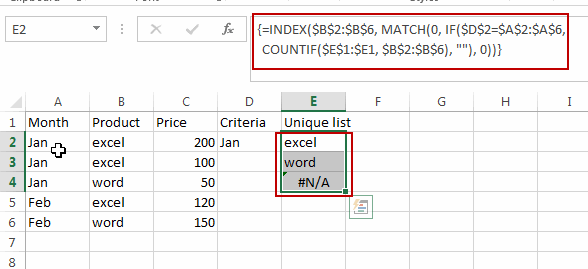# How to Extract a Unique List based on Criteria in Excel

This post will guide you how to extract unique values in a range based on one criteria with a formula in Excel. How do I create a unique distinct list based on one condition in Excel.

## Extract Unique List of Values based on Criteria

Assuming that you have a list of data in range A1:C6, in which contain price data for each product. And you want to extract a unique product list from this range and return non-duplicates that are unique based on the month value. For example, you want to extract a product name list based on Jan month.  You can use an Excel array formula based on the INDEX function, the MATCH function, the IF function, and the COUNTIF function to achieve the result. Here is the formula that it will extract the unique distinct product name list based on month value:

`=INDEX(\$B\$2:\$B\$6, MATCH(0, IF(\$D\$2=\$A\$2:\$A\$6, COUNTIF(\$E\$1:\$E1, \$B\$2:\$B\$6), ""), 0))`

Type this formula into Cell E2, and press Ctrl + Shift + Enter keys to change it as Array formula.  The drag the AutoFill handle until you get the #N/A value.You would notice that the unique product name list is extracted in column E.

Note: the range B2:B6 is a range that contains the unique product name list that you want to extract. A2:A6 is a range that contain criteria you want to base on.  Cell D2 contain one criteria that you want to use.

### Related Functions

• Excel COUNTIF function
The Excel COUNTIF function will count the number of cells in a range that meet a given criteria. This function can be used to count the different kinds of cells with number, date, text values, blank, non-blanks, or containing specific characters.etc.= COUNTIF (range, criteria)…
• Excel INDEX function
The Excel INDEX function returns a value from a table based on the index (row number and column number)The INDEX function is a build-in function in Microsoft Excel and it is categorized as a Lookup and Reference Function.The syntax of the INDEX function is as below:= INDEX (array, row_num,[column_num])…
• Excel MATCH function
The Excel MATCH function search a value in an array and returns the position of that item.The syntax of the MATCH function is as below:= MATCH  (lookup_value, lookup_array, [match_type])….
• Excel IF function
The Excel IF function perform a logical test to return one value if the condition is TRUE and return another value if the condition is FALSE. The IF function is a build-in function in Microsoft Excel and it is categorized as a Logical Function.The syntax of the IF function is as below:= IF (condition, [true_value], [false_value])….

### You might also like:

Sidebar